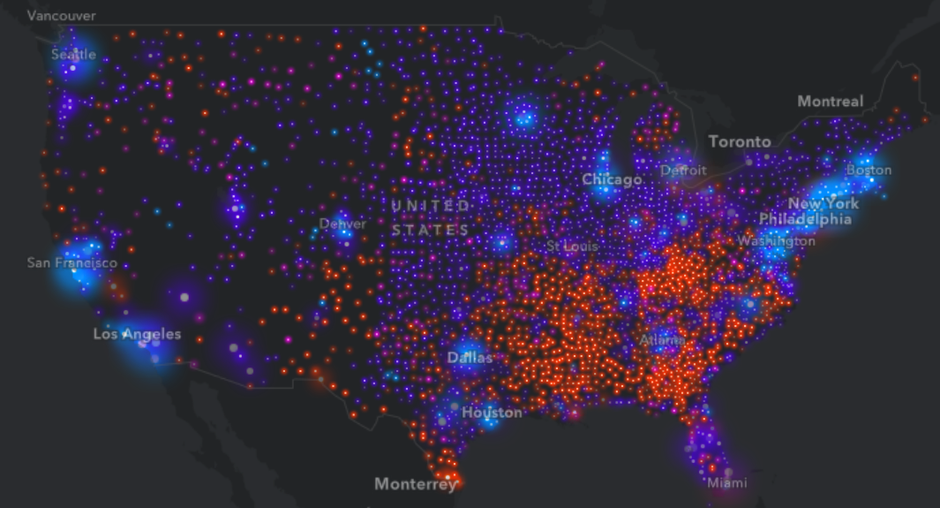### 可视化700年的利率下降情况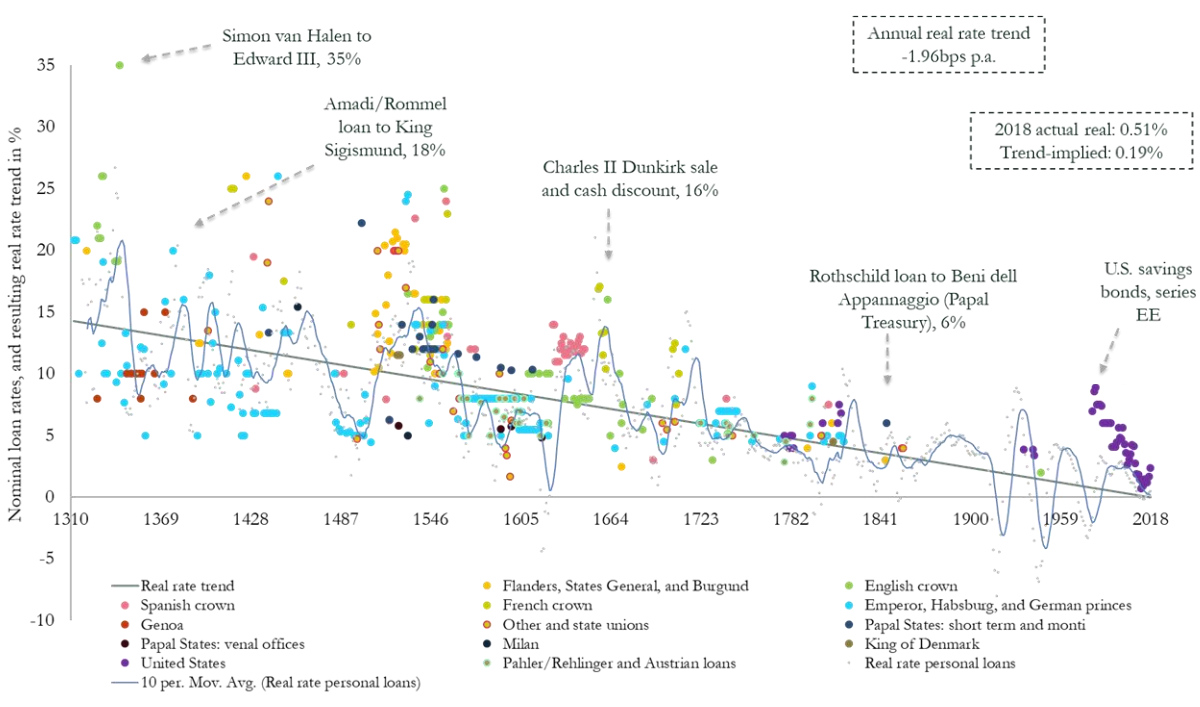### 因图生意：Famous Hope Quotes as Charts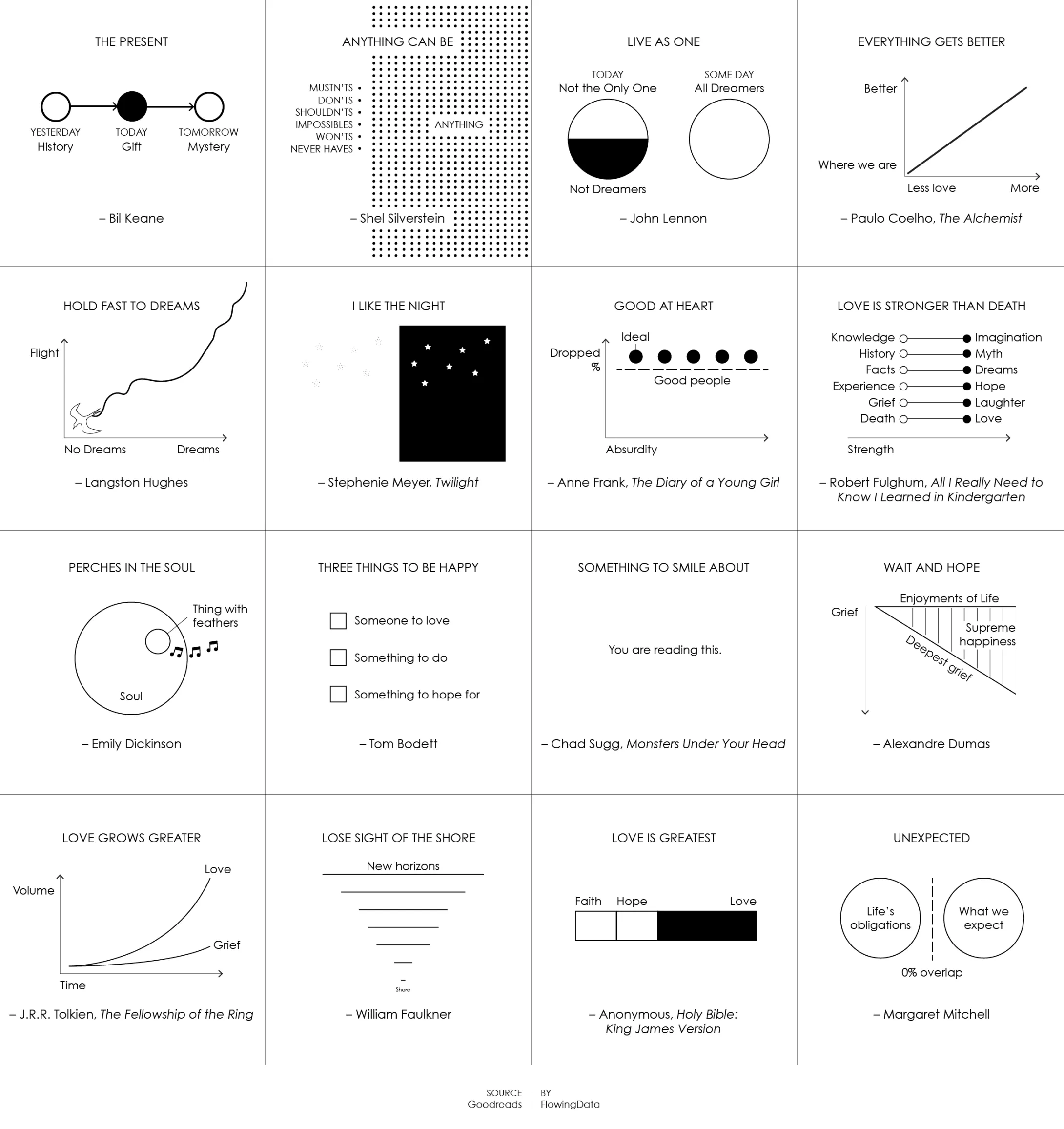### 手画线算法x0和y0代表开始点的坐标，xf，yf表示时间经过t = tf时候画到的位置。tf从开始画为 0 再到画完结束的时候为 2，这样画出来的线看起来是比较舒服的。并且为了使线更加的随机，我们在线的路径上面加上随机变化的量。

```// 计算贝塞尔曲线的中心点
const getControlPoint = (prev, next) => {
// 随机加上 -0.5 ~ 0.5的偏移量
const rdm = () => (Math.random() * 0.5 - 1);

const dx = rdm() * 10 - 5;
const dy = rdm() * 10 - 5;

return {
x: (prev.x + next.x) / 2 + dx,
y: (prev.y + next.y) / 2 + dy
};
}

// 通过主要算法得出点
function handLineAlg(sx, sy, fx, fy, t) {
const tau = t / 2.0;
const polyTerm = 15 * Math.pow(tau, 4) -
6 * Math.pow(tau, 5) -
10 * Math.pow(tau, 3);

return {
x: sx + (sx - fx) * polyTerm,
y: sy + (sy - fy) * polyTerm
};

}

// 得出G可以用来绘制的路径
const getHandLinePath = (startX, startY, endX, endY) => {
const [dx, dy] = [endX - startX, endY - startY];
// 按照线长短来改变控制点数
let dt = 1 / Math.sqrt(dx ** 2 + dy ** 2) * 200;
const path = [
['M', startX, startY]
];
let lastPoint = {
x: startX,
y: startY
};

// 根据论文里面，dt大于0.5
if (dt > 0.5) {
dt = 0.5
}

for (let t = 0; t <= 2.0; t += dt) {
const currentPoint = handLineAlg(startX, startY, endX, endY, t);
const squiggleControlPoint = getControlPoint(lastPoint, currentPoint);

path.push(['S', squiggleControlPoint.x, squiggleControlPoint.y,
currentPoint.x, currentPoint.y
])

lastPoint = currentPoint;
}

path.push(['L', endX, endY])

return path;
}```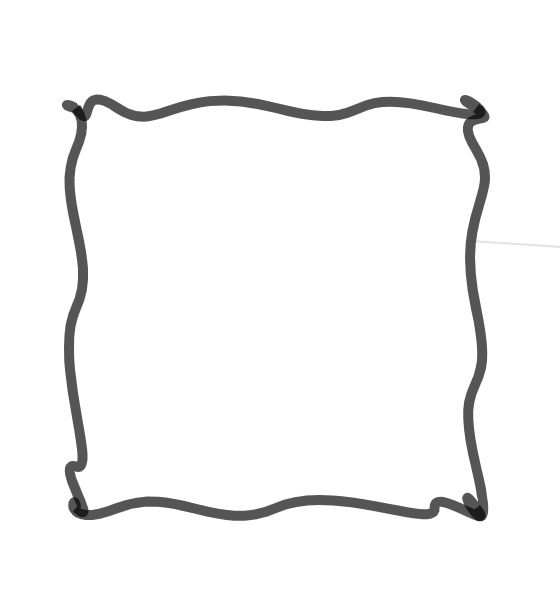### CO2 EMISSION VS. VULNERABILITY TO CLIMATE CHANGE, BY NATION (2010)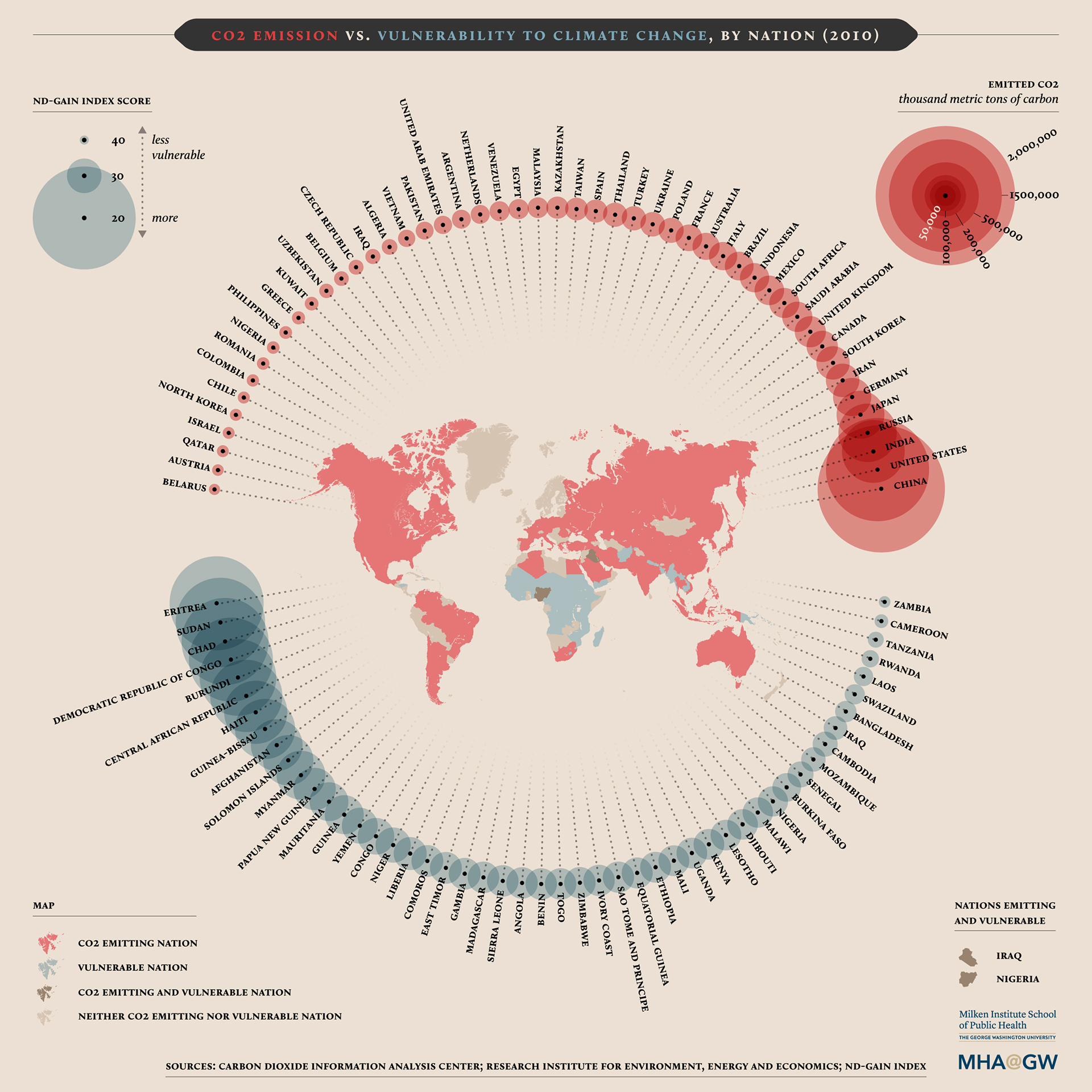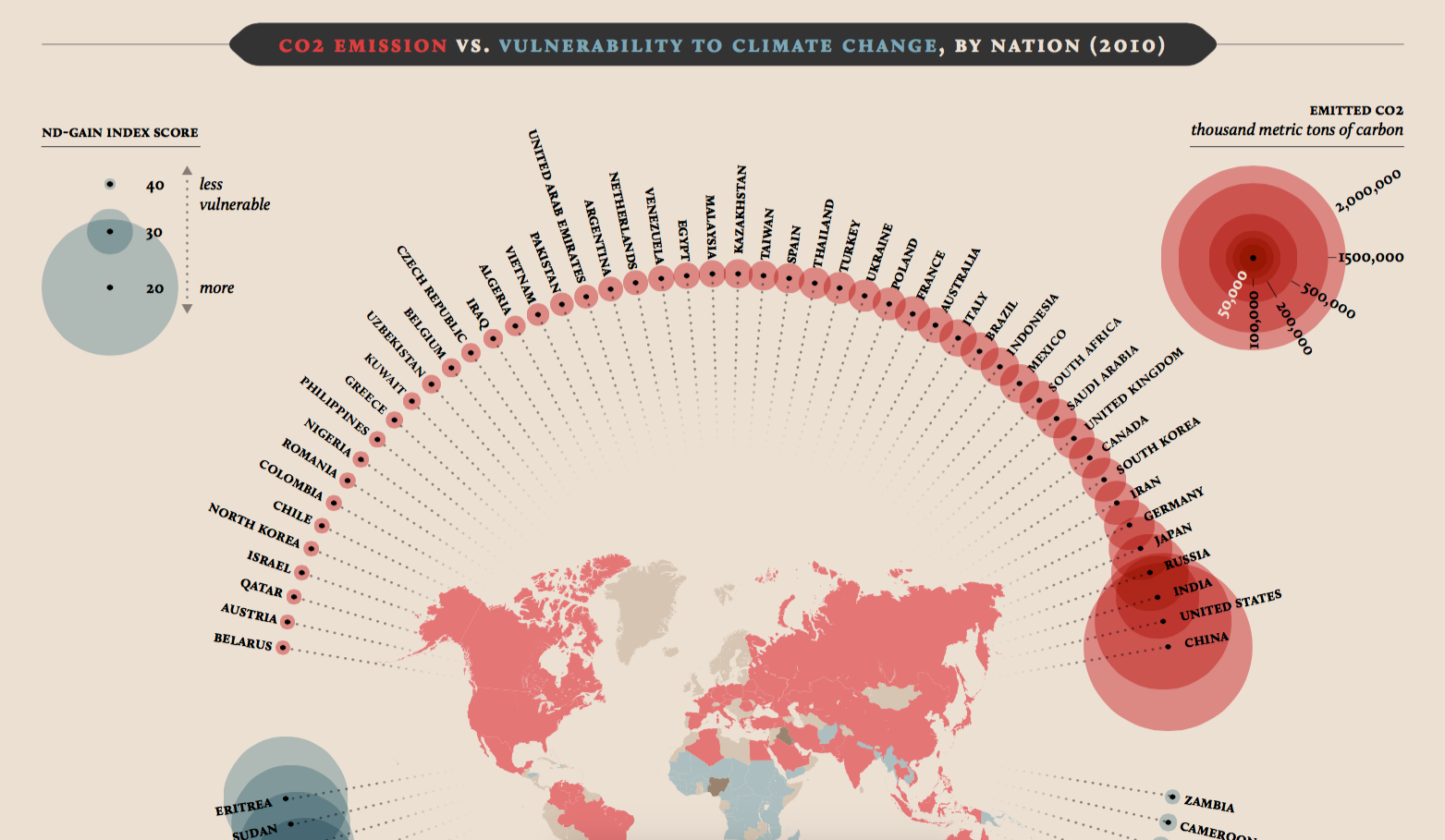### The COVID-19 Impact on App Popularity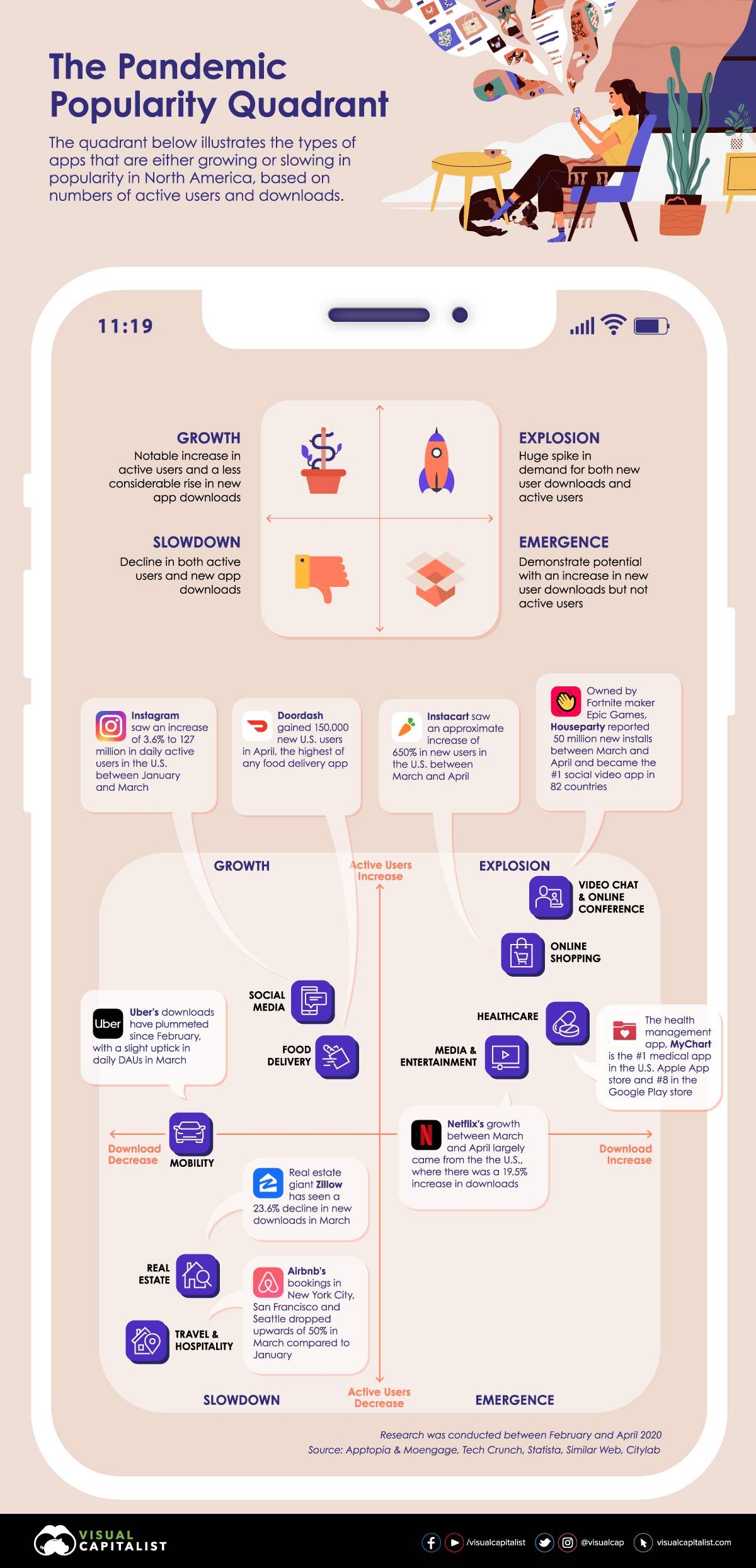### 观察巴黎不同类型道路的地图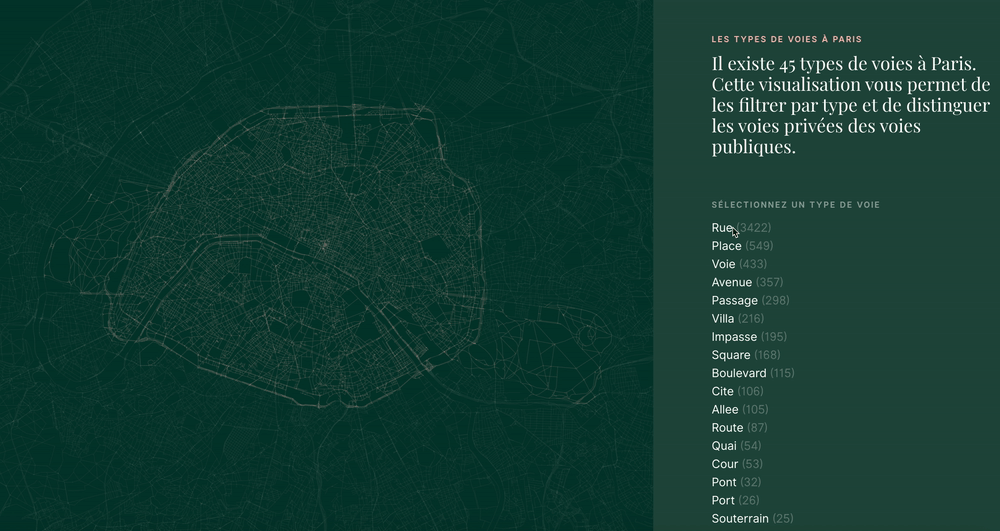### 通过可视化收入差异展现城市分化